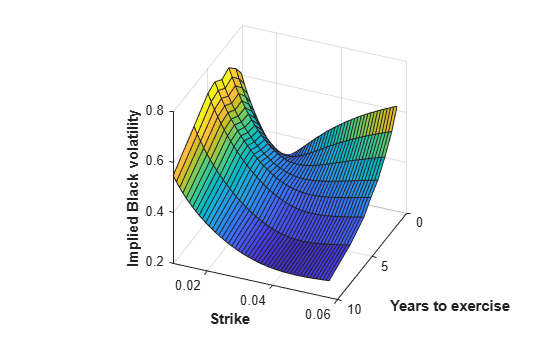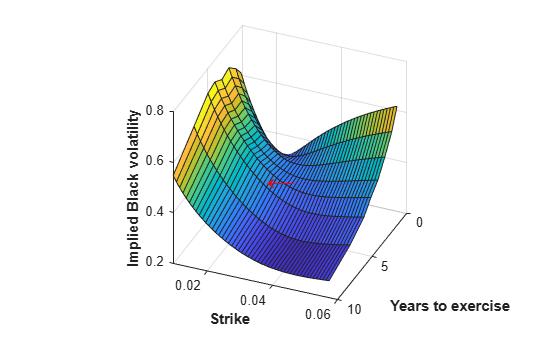# Price a Swaption Using the SABR Model

This example shows how to price a swaption using the SABR model. First, a swaption volatility surface is constructed from market volatilities. This is done by calibrating the SABR model parameters separately for each swaption maturity. The swaption price is then computed by using the implied Black volatility on the surface as an input to the `swaptionbyblk` function.

### Step 1. Load market swaption volatility data.

Load the market implied Black volatility data for swaptions.

```Settle = '12-Jun-2013'; ExerciseDates = {'12-Sep-2013';'12-Jun-2014';'12-Jun-2015';... '12-Jun-2016';'12-Jun-2017';'12-Jun-2018';'12-Jun-2020';... '12-Jun-2023'}; YearsToExercise = yearfrac(Settle, ExerciseDates, 1); NumMaturities = length(YearsToExercise); MarketVolatilities = [ ... 57.6 53.7 49.4 45.6 44.1 41.1 35.2 32.0 46.6 46.9 44.8 41.6 39.8 37.4 33.4 31.0 35.9 39.3 39.6 37.9 37.2 34.7 30.5 28.9 34.1 36.5 37.8 36.6 35.0 31.9 28.1 26.6 41.0 41.3 39.5 37.8 36.0 32.6 29.0 26.0 45.8 43.4 41.9 39.2 36.9 33.2 29.6 26.3 50.3 46.9 44.0 40.0 37.5 33.8 30.2 27.3]/100; MarketStrikes = [ ... 1.00 1.25 1.68 2.00 2.26 2.41 2.58 2.62; 1.50 1.75 2.18 2.50 2.76 2.91 3.08 3.12; 2.00 2.25 2.68 3.00 3.26 3.41 3.58 3.62; 2.50 2.75 3.18 3.50 3.76 3.91 4.08 4.12; 3.00 3.25 3.68 4.00 4.26 4.41 4.58 4.62; 3.50 3.75 4.18 4.50 4.76 4.91 5.08 5.12; 4.00 4.25 4.68 5.00 5.26 5.41 5.58 5.62]/100; CurrentForwardValues = MarketStrikes(4,:)```
```CurrentForwardValues = 1×8 0.0250 0.0275 0.0318 0.0350 0.0376 0.0391 0.0408 0.0412 ```
`ATMVolatilities = MarketVolatilities(4,:)`
```ATMVolatilities = 1×8 0.3410 0.3650 0.3780 0.3660 0.3500 0.3190 0.2810 0.2660 ```

The current underlying forward rates and the corresponding at-the-money volatilities across the eight swaption maturities are represented in the fourth rows of the two matrices.

### Step 2. Calibrate the SABR model parameters for each swaption maturity.

Using a model implemented in the function `blackvolbysabr`, a static SABR model, where the model parameters are assumed to be constant with respect to time, the parameters are calibrated separately for each swaption maturity (years to exercise) in a `for` loop. To better represent market at-the-money volatilities, the `Alpha` parameter values are implied by the market at-the-money volatilities (see "Method 2" for Calibrate the SABR Model).

Define the predetermined `Beta`, calibrate SABR model parameters for each swaption maturity and display calibrated parameters in a table.

```Beta = 0.5; Betas = repmat(Beta, NumMaturities, 1); Alphas = zeros(NumMaturities, 1); Rhos = zeros(NumMaturities, 1); Nus = zeros(NumMaturities, 1); options = optimoptions('lsqnonlin','Display','none'); for k = 1:NumMaturities % This function solves the SABR at-the-money volatility equation as a % polynomial of Alpha alpharoots = @(Rho,Nu) roots([... (1 - Beta)^2*YearsToExercise(k)/24/CurrentForwardValues(k)^(2 - 2*Beta) ... Rho*Beta*Nu*YearsToExercise(k)/4/CurrentForwardValues(k)^(1 - Beta) ... (1 + (2 - 3*Rho^2)*Nu^2*YearsToExercise(k)/24) ... -ATMVolatilities(k)*CurrentForwardValues(k)^(1 - Beta)]); % This function converts at-the-money volatility into Alpha by picking the % smallest positive real root atmVol2SabrAlpha = @(Rho,Nu) min(real(arrayfun(@(x) ... x*(x>0) + realmax*(x<0 || abs(imag(x))>1e-6), alpharoots(Rho,Nu)))); % Fit Rho and Nu (while converting at-the-money volatility into Alpha) objFun = @(X) MarketVolatilities(:,k) - ... blackvolbysabr(atmVol2SabrAlpha(X(1), X(2)), ... Beta, X(1), X(2), Settle, ExerciseDates(k), CurrentForwardValues(k), ... MarketStrikes(:,k)); X = lsqnonlin(objFun, [0 0.5], [-1 0], [1 Inf], options); Rho = X(1); Nu = X(2); % Get final Alpha from the calibrated parameters Alpha = atmVol2SabrAlpha(Rho, Nu); Alphas(k) = Alpha; Rhos(k) = Rho; Nus(k) = Nu; end CalibratedPrameters = array2table([Alphas Betas Rhos Nus],... 'VariableNames',{'Alpha' 'Beta' 'Rho' 'Nu'},... 'RowNames',{'3M into 10Y';'1Y into 10Y';... '2Y into 10Y';'3Y into 10Y';'4Y into 10Y';... '5Y into 10Y';'7Y into 10Y';'10Y into 10Y'})```
```CalibratedPrameters=8×4 table Alpha Beta Rho Nu ________ ____ ________ _______ 3M into 10Y 0.051947 0.5 0.39572 1.4146 1Y into 10Y 0.054697 0.5 0.2955 1.1257 2Y into 10Y 0.058433 0.5 0.24175 0.93463 3Y into 10Y 0.058484 0.5 0.20568 0.79647 4Y into 10Y 0.056054 0.5 0.13685 0.76993 5Y into 10Y 0.051072 0.5 0.060285 0.73595 7Y into 10Y 0.04475 0.5 0.083385 0.66341 10Y into 10Y 0.044548 0.5 0.02261 0.49487 ```

### Step 3. Construct a volatility surface.

Use the calibrated model to compute new volatilities at any strike value to produce a smooth smile for a given maturity. This can be repeated for each maturity to form a volatility surface

Compute volatilities using the calibrated models for each maturity and plot the volatility surface.

```PlottingStrikes = (0.95:0.1:5.8)'/100; ComputedVols = zeros(length(PlottingStrikes), NumMaturities); for k = 1:NumMaturities ComputedVols(:,k) = blackvolbysabr(Alphas(k), Betas(k), Rhos(k), Nus(k), Settle, ... ExerciseDates(k), CurrentForwardValues(k), PlottingStrikes); end figure; surf(YearsToExercise, PlottingStrikes, ComputedVols); xlim([0 10]); ylim([0.0095 0.06]); zlim([0.2 0.8]); view(113,32); set(gca, 'Position', [0.13 0.11 0.775 0.815], ... 'PlotBoxAspectRatioMode', 'manual'); xlabel('Years to exercise', 'Fontweight', 'bold'); ylabel('Strike', 'Fontweight', 'bold'); zlabel('Implied Black volatility', 'Fontweight', 'bold');```Note, in this volatility surface, the smiles tend to get flatter for longer swaption maturities (years to exercise). This is consistent with the `Nu` parameter values tending to decrease with swaption maturity, as shown previously in the table for `CalibratedPrameters`.

### Step 4. Use `swaptionbyblk` to price a swaption.

Use the volatility surface to price a swaption that matures in five years. Define a swaption (for a 10-year swap) that matures in five years and use the interest-rate term structure at the time of the swaption `Settle` date to define the `RateSpec`. Use the `RateSpec` to compute the current forward swap rate using the `swapbyzero` function. Compute the SABR implied Black volatility for this swaption using the `blackvolbysabr` function (and it is marked with a red arrow in the figure that follows). Price the swaption using the SABR implied Black volatility as an input to the `swaptionbyblk` function.

```% Define the swaption SwaptionSettle = '12-Jun-2013'; SwaptionExerciseDate = '12-Jun-2018'; SwapMaturity = '12-Jun-2028'; Reset = 1; OptSpec = 'call'; Strike = 0.0263; % Define RateSpec ValuationDate = '12-Jun-2013'; EndDates = {'12-Jul-2013';'12-Sep-2013';'12-Dec-2013';'12-Jun-2014';... '12-Jun-2015';'12-Jun-2016';'12-Jun-2017';'12-Jun-2018';... '12-Jun-2019';'12-Jun-2020';'12-Jun-2021';'12-Jun-2022';... '12-Jun-2023';'12-Jun-2025';'12-Jun-2028';'12-Jun-2033'}; Rates = [0.2 0.3 0.4 0.7 0.5 0.7 1.0 1.4 1.7 1.9 ... 2.1 2.3 2.5 2.8 3.1 3.3]'/100; Compounding = 1; RateSpec = intenvset('ValuationDate', ValuationDate, 'StartDates', ValuationDate, ... 'EndDates', EndDates, 'Rates', Rates, 'Compounding', Compounding)```
```RateSpec = struct with fields: FinObj: 'RateSpec' Compounding: 1 Disc: [16x1 double] Rates: [16x1 double] EndTimes: [16x1 double] StartTimes: [16x1 double] EndDates: [16x1 double] StartDates: 735397 ValuationDate: 735397 Basis: 0 EndMonthRule: 1 ```
```% Use swapbyzero LegRate = [NaN 0]; % To compute the forward swap rate, set the coupon rate to NaN. [~, CurrentForwardSwapRate] = swapbyzero(RateSpec, LegRate, SwaptionSettle, SwapMaturity,... 'StartDate', SwaptionExerciseDate); % Use blackvolbysabr SABRBlackVolatility = blackvolbysabr(Alphas(6), Betas(6), Rhos(6), Nus(6), SwaptionSettle, ... SwaptionExerciseDate, CurrentForwardSwapRate, Strike)```
```SABRBlackVolatility = 0.3932 ```
```text (YearsToExercise(6), Strike, SABRBlackVolatility, '\leftarrow',... 'Color', 'r', 'FontWeight', 'bold', 'FontSize', 22);``````% Use swaptionbyblk Price = swaptionbyblk(RateSpec, OptSpec, Strike, SwaptionSettle, SwaptionExerciseDate, ... SwapMaturity, SABRBlackVolatility, 'Reset', Reset)```
```Price = 14.2403 ```

 Hagan, P. S., Kumar, D., Lesniewski, A. S. and Woodward, D. E., “Managing Smile Risk,” Wilmott Magazine, 2002.

 West, G., “Calibration of the SABR Model in Illiquid Markets,” Applied Mathematical Finance, 12(4), pp. 371–385, 2004.